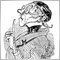# Ordertype based on indicator64

Hello, I need your help. I want that the ea open an order in the direction which the ea has give his signal but it doesent worked for any reason. Can someone help me?

The EA onTick

```void OnTick()
{

if(check == false)
{
double Equity = AccountEquity();
Verlust = -Equity*RiskPercent*0.01;
Gewinn =  Equity*RewardPercent*0.01;

double freeMargin = AccountFreeMargin();
halfMargin = freeMargin/4;
rechnung = halfMargin*750;
lots = rechnung/100000;

check = true;
}

if(check == true)
{
down=iCustom(NULL,0,"ASCTrend",1,0);
up=iCustom(NULL,0,"ASCTrend",0,0);

if(OrdersTotal() == 0 && check == true)
{
{
opensell();
check=false;
}// if(downtrend!=EMPTY_VALUE)

{
check=false;
}// else(uptrend!=EMPTY_VALUE)

}// if(OrdersTotal()==0)

...
...
...
}//void OnTick()```

The indicator

ASCTrend

```//+------------------------------------------------------------------+
//| ASCTrend1sig_noSound.mq4
//| Ramdass - Conversion only
//+------------------------------------------------------------------+

#property indicator_chart_window
#property indicator_buffers 2
#property indicator_color1 Magenta
#property indicator_color2 Aqua

//---- input parameters
extern int RISK=1;
extern int CountBars=500;

//---- buffers
double val1[];
double val2[];

//+------------------------------------------------------------------+
//| Custom indicator initialization function                         |
//+------------------------------------------------------------------+
int init()
{
//---- indicator line
IndicatorBuffers(2);
SetIndexStyle(0,DRAW_ARROW);
SetIndexArrow(0,234);
SetIndexStyle(1,DRAW_ARROW);
SetIndexArrow(1,233);
SetIndexBuffer(0,val1);
SetIndexBuffer(1,val2);

//----
return(0);
}
//+------------------------------------------------------------------+
//| ASCTrend1sig                                                     |
//+------------------------------------------------------------------+
int start()
{
if (CountBars>=1000) CountBars=950;
SetIndexDrawBegin(0,Bars-CountBars+11+1);
SetIndexDrawBegin(1,Bars-CountBars+11+1);
int i,shift,counted_bars=IndicatorCounted();
int Counter,i1,value10,value11;
double value1,x1,x2;
double value2,value3;
double TrueCount,Range,AvgRange,MRO1,MRO2;
double Table_value2;

value10=3+RISK*2;
x1=67+RISK;
x2=33-RISK;
value11=value10;
//----
if(Bars<=11+1) return(0);
//---- initial zero
if(counted_bars<11+1)
{
for(i=1;i<=0;i++) val1[CountBars-i]=0.0;
for(i=1;i<=0;i++) val2[CountBars-i]=0.0;
}
//----
shift=CountBars-11-1;
while(shift>=0)
{

Counter=shift;
Range=0.0;
AvgRange=0.0;
for (Counter=shift; Counter<=shift+9; Counter++) AvgRange=AvgRange+MathAbs(High[Counter]-Low[Counter]);

Range=AvgRange/10;
Counter=shift;
TrueCount=0;
while (Counter<shift+9 && TrueCount<1)
{if (MathAbs(Open[Counter]-Close[Counter+1])>=Range*2.0) TrueCount=TrueCount+1;
Counter=Counter+1;
}
if (TrueCount>=1) {MRO1=Counter;} else {MRO1=-1;}
Counter=shift;
TrueCount=0;
while (Counter<shift+6 && TrueCount<1)
{if (MathAbs(Close[Counter+3]-Close[Counter])>=Range*4.6) TrueCount=TrueCount+1;
Counter=Counter+1;
}
if (TrueCount>=1) {MRO2=Counter;} else {MRO2=-1;}
if (MRO1>-1) {value11=3;} else {value11=value10;}
if (MRO2>-1) {value11=4;} else {value11=value10;}
value2=100-MathAbs(iWPR(NULL,0,value11,shift)); // PercentR(value11=9)
Table_value2[shift]=value2;
val1[shift]=0;
val2[shift]=0;
value3=0;
if (value2<x2)
{i1=1;
while (Table_value2[shift+i1]>=x2 && Table_value2[shift+i1]<=x1){i1++;}
if (Table_value2[shift+i1]>x1)
{
value3=High[shift]+Range*0.5;
val1[shift]=value3;
}
}
if (value2>x1)
{i1=1;
while (Table_value2[shift+i1]>=x2 && Table_value2[shift+i1]<=x1){i1++;}
if (Table_value2[shift+i1]<x2)
{
value3=Low[shift]-Range*0.5;
val2[shift]=value3;
}
}

shift--;
}

return(0);
}
//+------------------------------------------------------------------+

```Moderator
12290

At first I would check the values you receive from your indicator:

```if(check == true)
{
down=iCustom(NULL,0,"ASCTrend",1,0);
up=iCustom(NULL,0,"ASCTrend",0,0);
if ( (down>0 && down<EMPTY_VALUE) || (up>0 && up<EMPTY_VALUE) )
Comment(TimeToString(TimeCurrent()),"  down: ",DoubleToStr(down,Digits),"  up: ",DoubleToStr(up,Digits))

(There may by typos!)

Use M1 then you don't have to wait to long!64

Thank you for helping. It comment the price of the drawn arrow. The problem is that the ea is opening at the moment multiple orders and I want that it opens only one order with 1 signal. Do you have an idea ?Moderator
12290

1) save the ticket number in a global-variable e.g. int Ticket = -1; // no order

2)  if(Ticket<0) Ticket = OrderSend(..);

3) if (doClose) { closeOrder(..); Ticket=-1; ..}

s.th. like that?1537

Hello,

` down = iCustom(NULL,0,"ASCTrend1sig_noSound", RISK, CountBars,1,0);`25838

1. ```   if(down!=EMPTY_VALUE && opentrade ==false)
{
opensell();
```
You are checking against EMPTY_VALUE.
2. ```        val1[shift]=0;
val2[shift]=0;```
Indicator always sets the buffers to zero or a value.
3. Therefor, you ALWAYS open a sell.
4. You would have seen this had you opened the data window (control-d) and looked at the indicator values.64

First of all, thank you guys for helping.

@WHRoeder: So how can I fix that problem?

```if(down>0)
{
opensell();

}// if(downtrend!=EMPTY_VALUE)

else if(up>0)
{

}// else(uptrend!=EMPTY_VALUE)```

Now the ea have to check if the value is bigger then 0 but the problem is still there, the ea open multiple trades on the same signal1537

``` if(down!=0.0 && opentrade ==false)
{
opensell();```64

still not working...Moderator
32147

albo111:
still not working...64

Good idea, that is the current version of the code

```void OnTick()
{

if(check == false)
{
double Equity = AccountEquity();
Verlust = -Equity*RiskPercent*0.01;
Gewinn =  Equity*RewardPercent*0.01;

double freeMargin = AccountFreeMargin();
halfMargin = freeMargin/4;
rechnung = halfMargin*750;
lots = rechnung/100000;

check = true;
}

if(check == true && OrdersTotal()==0)
{
down=iCustom(NULL,0,"ASCTrend",1,0);
up=iCustom(NULL,0,"ASCTrend",0,0);

if ( (down>0 && down<EMPTY_VALUE) || (up>0 && up<EMPTY_VALUE) )

Comment(TimeToString(TimeCurrent()),"  down: ",DoubleToStr(down,Digits),"  up: ",DoubleToStr(up,Digits));

if(OrdersTotal() == 0 && check == true)
{
if(down>0 && down!=olddown)
{
opensell();

}// if(downtrend!=EMPTY_VALUE)

else if(up>0 &&up!=oldup)
{

}// else(uptrend!=EMPTY_VALUE)

}// if(OrdersTotal()==0)

if(OrdersTotal()>0)
{
if(AccountProfit()>=Gewinn)
{
//oldup = up;
olddown = down;

closeallorders();

}// if(AccountProfit()>=Gewinn)

if(AccountProfit()<=Verlust)
{
//oldup = up;
olddown = down;

closeallorders();

}// if(AccountProfit()<=Verlust)

}// if(OrdersTotal()>0)

}//void OnTick()```